#### Tuesday - February 2nd### Tuesday, February 2nd

All times are in Central European Time (CET). Underlined authors are presenting the talks.
Click on the talk title to access the recording.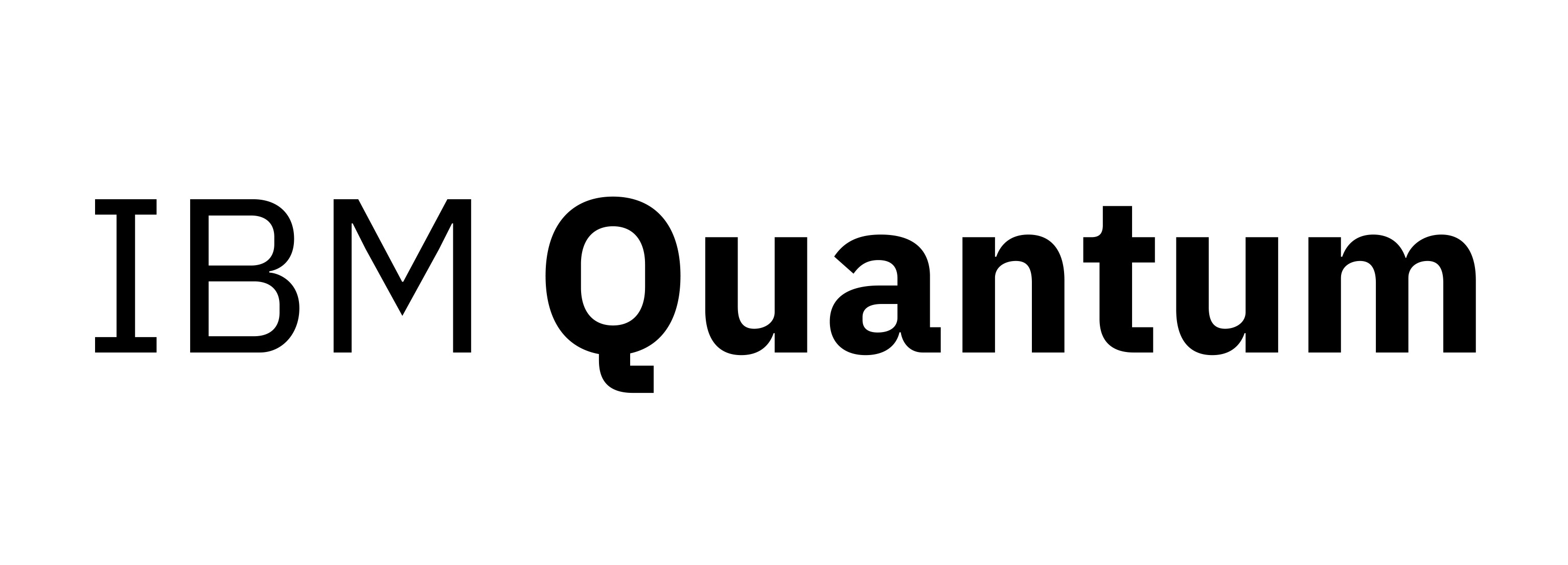Sessions on Tuesday are sponsored by IBM Quantum.

### Monday | Wednesday | Thursday | Friday

##### 08:00 - 8:30 | Breakfast Club - Networking

Find out who else is here and discuss via video chat in a casual atmosphere different serious (Quantum Computing: hype or hope?) and not so serious topics (What’s your favourite Bavarian dish?). Join the "Social activities" tab in Meetanyway. Topics change daily. You can join and leave tables as you wish.

### Session 1A

Main stage (A)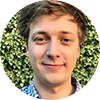Authors: Sam Roberts and Dominic Williamson

Abstract

Affiliations: PsiQuantum | Stanford University

Extended abstract

Abstract
We present a scheme for universal topological quantum computation based on Clifford complete braiding and fusion of symmetry defects in the 3-Fermion anyon theory, supplemented with magic state injection. We formulate a fault-tolerant measurement-based realisation of this computational scheme on the lattice using ground states of the Walker--Wang model for the 3-Fermion anyon theory with symmetry defects. The Walker--Wang measurement-based topological quantum computation paradigm that we introduce provides a general construction of computational resource states with thermally stable symmetry-protected topological order. We also demonstrate how symmetry defects of the 3-Fermion anyon theory can be realized in a 2D subsystem code due to Bombin -- pointing to an alternative implementation of our 3-Fermion defect computation scheme via code deformations.9:00 - 9:30
Entanglement bootstrap program

Authors: Bowen Shi, Kohtaro Kato and Isaac Kim

Abstract

Affiliations: University of California San Diego | Osaka University | The University of Sydney

Extended abstract

Abstract
We introduce the entanglement bootstrap program, a powerful new approach to study topologically ordered quantum many-body systems. In this program, we posit that local reduced density matrices of the underlying system obey a set of simple constraints that are motivated by the entanglement entropy calculations in the literature. We show that these constraints lead to a highly nontrivial set of identities that can be interpreted as the basic axioms of the emergent theory describing the topological charges in such systems. The surprising nature of our work lies in the fact that these basic axioms of the emergent theory, which are typically assumed in the literature, can actually be derived from a simple entanglement property of the ground state. Furthermore, we apply this line of reasoning to systems with gapped domain walls and derive a hitherto unknown set of topological charges and identities that those topological charges need to satisfy. Thus, our work establishes a deep connection between entanglement and the exotic behaviors of topological charges in topologically ordered systems.Authors: Kianna Wan, Soonwon Choi, Isaac Kim, Noah Shutty and Patrick Hayden

Abstract

Affiliations: Stanford University | University of California, Berkeley | The University of Sydney | Stanford University | Stanford University

Extended abstract

Abstract
With gate error rates in multiple technologies now below the threshold required for fault-tolerant quantum computation, the major remaining obstacle to useful quantum computation is scaling, a challenge greatly amplified by the huge overhead imposed by quantum error correction itself. We propose a fault-tolerant quantum computing scheme that can nonetheless be assembled from a small number of experimental components, potentially dramatically reducing the engineering challenges associated with building a large-scale fault-tolerant quantum computer. Our scheme has a threshold of $0.39\%$ for depolarising noise, assuming that memory errors are negligible. In the presence of memory errors, the logical error rate decays exponentially with $\sqrt{T/\tau}$, where $T$ is the memory coherence time and $\tau$ is the timescale for elementary gates. Our approach is based on a novel procedure for fault-tolerantly preparing three-dimensional cluster states using a single actively controlled qubit and a pair of delay lines. Although a circuit-level error may propagate to a high-weight error, the effect of this error on the prepared state is always equivalent to that of a constant-weight error. We describe how the requisite gates can be implemented using existing technologies in quantum photonic and phononic systems. With continued improvements in only a few components, we expect these systems to be promising candidates for demonstrating fault-tolerant quantum computation with a comparatively modest experimental effort.

### Session 1B

Stage B8:30 - 9:00

On the entropic convergence of quantum Gibbs samplers

Authors: Cambyse Rouzé, Ivan Bardet, Ángela Capel and Daniel Stilck França

Abstract

Affiliations: Technische Universität München | INRIA Paris | Technische Universität München | University of Copenhagen

Extended abstract

Abstract
Given a uniform, frustration-free family of local Lindbladians defined on a quantum lattice spin system in any spatial dimension, we prove a strong exponential convergence in relative entropy of the system to equilibrium under a condition of spatial mixing of the stationary Gibbs states and the rapid decay of the relative entropy on finite-size blocks. Our result leads to the first examples of the positivity of the modified logarithmic Sobolev inequality for quantum lattice spin systems independently of the system size. Moreover, we show that our notion of spatial mixing is a consequence of the recent quantum generalization of Dobrushin and Shlosman's complete analyticity of the free-energy at equilibrium. The latter typically holds above a critical temperature $T_c$. Our results have wide applications in quantum information processing. As an illustration, we discuss three of them: first, using techniques of quantum optimal transport, we show that a quantum annealer subject to a finite range classical noise will output an energy close to that of the fixed point after constant annealing time. Second, we prove a finite blocklength refinement of the quantum Stein lemma for the task of asymmetric discrimination of two Gibbs states of commuting Hamiltonians satisfying our conditions. In the same setting, our results imply the existence of a local quantum circuit of logarithmic depth to prepare Gibbs states of a class of commuting Hamiltonians.Authors: Tomotaka Kuwahara, Alvaro Alhambra and Anurag Anshu

Abstract

Affiliations: RIKEN Center for Advanced Intelligence Project | Max Planck Institute for Quantum Optics | University of California, Berkeley

Extended abstract

Abstract
One of the most fundamental problems in quantum many-body physics is the characterization of correlations among thermal states. Of particular relevance is the thermal area law, which justifies the tensor network approximations to thermal states with a bond dimension growing polynomially with the system size. In the regime of sufficiently low temperatures, which is particularly important for practical applications, the existing techniques do not yield optimal bounds. Here, we propose a new thermal area law that holds for generic many-body systems on lattices. We improve the temperature dependence from the original O(β)to ̃O(β^2/3), thereby suggesting diffusive propagation of entanglement by imaginary time evolution. This qualitatively differs from the real-time evolution which usually induces linear growth of entanglement. We also prove analogous bounds for the Rényi entanglement of purification and the entanglement of formation. Our analysis is based on a polynomial approximation to the exponential function which provides a relationship between the imaginary-time evolution and random walks. Moreover, for one-dimensional (1D) systems with n spins, we prove that the Gibbs state is well-approximated by a matrix product operator with a sublinear bond dimension of exp( ̃O(βlog(n))). This allows us to rigorously establish, for the first time, a quasi-linear time classical algorithm for constructing an MPS representation of 1D quantum Gibbs states at arbitrary temperatures ofβ=o(log(n)). Our new technical ingredient is a block decomposition of the Gibbs state, that bears resemblance to the decomposition of real-time evolution given by Haah et al., FOCS’18.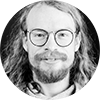Authors: Daniel Ranard, Freek Witteveen and Michael Walter

Abstract

Affiliations: Stanford University | University of Amsterdam | University of Amsterdam

Abstract
Unitary dynamics with a strict causal cone (or "light cone") have been studied extensively, under the name of locality preserving unitaries (LPUs) or quantum cellular automata. In particular, LPUs in one dimension have been completely classified by an index theory. Physical systems often exhibit only approximate causal cones; Hamiltonian evolutions on the lattice satisfy Lieb-Robinson bounds rather than strict locality. This motivates us to study approximately locality preserving unitaries (ALPUs). We show that the index theory is robust and completely extends to one-dimensional ALPUs. As a consequence, we achieve a converse to the Lieb-Robinson bounds: any ALPU of index zero can be exactly generated by some time-dependent, quasi-local Hamiltonian in constant time. For the special case of finite chains with open boundaries, any unitary satisfying the Lieb-Robinson bound may be generated by such a Hamiltonian. We also discuss some results on the stability of operator algebras which may be of independent interest.

### Session 1C

Stage C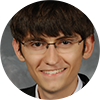8:30 - 9:00
The Quantum Supremacy Tsirelson Inequality

Authors: William Kretschmer

Abstract

Affiliations: University of Texas at Austin

Extended abstract

Abstract
A leading proposal for verifying near-term quantum supremacy experiments on noisy random quantum circuits is linear cross-entropy benchmarking. For a quantum circuit $C$ on $n$ qubits and a sample $z \in \{0,1\}^n$, the benchmark involves computing $|\langle z|C|0^n \rangle|^2$, i.e. the probability of measuring $z$ from the output distribution of $C$ on the all zeros input. Under a strong conjecture about the classical hardness of estimating output probabilities of quantum circuits, no polynomial-time classical algorithm given $C$ can output a string $z$ such that $|\langle z|C|0^n\rangle|^2$ is substantially larger than $\frac{1}{2^n}$ (Aaronson and Gunn, 2019). On the other hand, for a random quantum circuit $C$, sampling $z$ from the output distribution of $C$ achieves $|\langle z|C|0^n\rangle|^2 \approx \frac{2}{2^n}$ on average (Arute et al., 2019). In analogy with the Tsirelson inequality from quantum nonlocal correlations, we ask: can a polynomial-time quantum algorithm do substantially better than $\frac{2}{2^n}$? We study this question in the query (or black box) model, where the quantum algorithm is given oracle access to $C$. We show that, for any $\varepsilon \ge \frac{1}{\mathrm{poly}(n)}$, outputting a sample $z$ such that $|\langle z|C|0^n\rangle|^2 \ge \frac{2 + \varepsilon}{2^n}$ on average requires at least $\Omega\left(\frac{2^{n/4}}{\mathrm{poly}(n)}\right)$ queries to $C$, but not more than $O\left(2^{n/3}\right)$ queries to $C$, if $C$ is either a Haar-random $n$-qubit unitary, or a canonical state preparation oracle for a Haar-random $n$-qubit state. We also show that when $C$ samples from the Fourier distribution of a random Boolean function, the naive algorithm that samples from $C$ is the optimal 1-query algorithm for maximizing $|\langle z|C|0^n\rangle|^2$ on average.Authors: Justin Holmgren and Ran Raz

Abstract

Affiliations: NTT Research | Princeton University

Abstract
We prove that parallel repetition of the (3-player) GHZ game reduces the value of the game polynomially fast to~0. That is, the value of the GHZ game repeated in parallel $t$ times is at most $t^{-\Omega(1)}$. Previously, only a bound of $\approx \frac{1}{\alpha(t)}$, where $\alpha$ is the inverse Ackermann function, was known (Verbitsky '96). The GHZ game was recently identified by Dinur, Harsha, Venkat and Yuen as a multi-player game where all existing techniques for proving strong bounds on the value of the parallel repetition of the game fail. Indeed, to prove our result we use a completely new proof technique. Dinur, Harsha, Venkat and Yuen speculated that progress on bounding the value of the parallel repetition of the GHZ game may lead to further progress on the general question of parallel repetition of multi-player games. They suggested that the strong correlations present in the GHZ question distribution represent the hardest instance'' of the multi-player parallel repetition problem. Another motivation for studying the parallel repetition of the GHZ game comes from the field of quantum information. The GHZ game, first introduced by Greenberger, Horne and Zeilinger, is a central game in the study of quantum entanglement and has been studied in numerous works. For example, it is used for testing quantum entanglement and for device-independent quantum cryptography. In such applications a game is typically repeated to reduce the probability of error, and hence bounds on the value of the parallel repetition of the game may be useful.Authors: Satvik Singh and Ion Nechita

Abstract

Affiliations: Department of Physical Sciences, Indian Institute of Science Education and Research, Mohali | Université de Toulouse

Extended abstract

Abstract
In the rapidly developing field of Quantum technologies, the task of entanglement distribution between two parties occupies a central stage in many important protocols. However, as the distance between the two parties increases, the error probability in any transmission channel gets larger, resulting in degradation of the quality of the distributed entanglement. To overcome this problem, quantum repeater devices are used. The basic idea of such a device is to split up the long transmission channel into shorter manageable segments, each of which can be provided with high fidelity entangled states. Then, the well-known entanglement swapping technique can be used to transfer the entanglement from the intermediate segments to the ends of the long channel. A key conjecture in this regard was proposed by M. Christandl, which states that all PPT entangled states are useless from the perspective of repeater devices, since the swapping of entanglement in such states inevitably leads to a separable state. The conjecture admits an equivalent formulation in terms of linear maps, where it amounts to saying that the composition of any two PPT maps (these are the maps which are both completely positive and completely copositive) is entanglement-breaking. In the present work, we prove that this conjecture holds for all linear maps which are covariant under the diagonal unitary group’s action. Many salient examples like the Choi-type maps, Schur multipliers, Classical maps, etc. lie in this class. Our proof relies on a generalization of the matrix-theoretic notion of factor width for pairwise completely positive matrices, as well as on our previous characterization of the aforementioned class of maps. Hence, in a nutshell, our research proves the unsuitability of a large class of states from the perspective of repeater protocols and thus significantly contributes to the solution of a long-standing open problem in quantum information theory.##### 10:00 - 10:30 | The XZZX surface code

Authors: Pablo Bonilla Ataides, David Tuckett, Stephen Bartlett, Steven Flammia and Benjamin Brown

ABSTRACT

Affiliation: University of Sydney | University of Sydney | The University of Sydney | AWS | University of Sydney

Extended abstract

Abstract
We show that a variant of the surface code---the XZZX code---offers remarkable performance for fault-tolerant quantum computation. The error threshold of this code matches what can be achieved with random codes (hashing) for \emph{every} single-qubit Pauli noise channel; it is the first explicit code shown to have this universal property. We present numerical evidence that the threshold even exceeds this hashing bound for an experimentally relevant range of noise parameters. Focusing on the common situation where qubit dephasing is the dominant noise, we show that this code has a practical, high-performance decoder and surpasses all previously known thresholds in the realistic setting where syndrome measurements are unreliable. We go on to demonstrate the favorable sub-threshold resource scaling that can be obtained by specializing a code to exploit structure in the noise. We show that it is possible to maintain all of these advantages when we perform fault-tolerant quantum computation. We finally suggest some small-scale experiments that could exploit noise bias to reduce qubit overhead in two-dimensional architectures. The complete version of this paper can be found at https://arxiv.org/abs/2009.07851.

##### 10:30 - 11:00 | Round tables

At the end of the session, you can meet with the speakers directly. Round tables take place in parallel, each speaker having their own meeting.

##### 11:30 - 13:30 | Poster Session B

Posters in Rooms B.1 to B.4 are presenting. Visit the 3D poster rooms and use the "Video chat" button under each poster to engage with the poster representative during this time. This is linked to their poster in MeetAnyway, therefore access is possible from either platform.

##### 14:30 - 15:00 | Networking

Find out who else is here and discuss via video chat in a casual atmosphere different serious (Quantum Computing: hype or hope?) and not so serious topics (What’s your favourite Bavarian dish?). Join the "Social activities" tab in MeetAnyway. Topics change daily. You can join and leave tables as you wish.

### Session 2A

Main stage (A)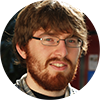15:00 - 15:30
Fault-tolerant coding for quantum communication

Authors: Alexander Müller-Hermes and Matthias Christandl

Abstract

Affiliations: Claude Bernard University of Lyon 1 | University of Copenhagen

Extended abstract

Abstract
Designing encoding and decoding circuits to reliably send messages over many uses of a noisy channel is a central problem in communication theory. When studying the optimal transmission rates achievable with asymptotically vanishing error it is usually assumed that these circuits can be implemented using noise-free gates. While this assumption is satisfied for classical machines in many scenarios, it is not expected to be satisfied in the near term future for quantum machines where decoherence leads to faults in the quantum gates. As a result, fundamental questions regarding the practical relevance of quantum channel coding remain open. By combining techniques from fault-tolerant quantum computation with techniques from quantum communication, we initiate the study of these questions. We introduce fault-tolerant versions of quantum capacities quantifying the optimal communication rates achievable with asymptotically vanishing total error when the encoding and decoding circuits are affected by gate errors with small probability. Our main results are threshold theorems for the classical and quantum capacity: For every quantum channel $T$ and every $\epsilon>0$ there exists a threshold $p(\epsilon,T)$ for the gate error probability below which rates larger than $C-\epsilon$ are fault-tolerantly achievable with vanishing overall communication error, where $C$ denotes the usual capacity. Our results are not only relevant in communication over large distances, but also on-chip, where distant parts of a quantum computer might need to communicate under higher levels of noise than affecting the local gates.

### Session 2B

Stage B15:00 - 15:30
Emergent classicality in multipartite channels

Authors: Daniel Ranard and Xiao-Liang Qi

Abstract

Affiliations: Stanford University | Stanford University

Extended abstract

Abstract
In a quantum measurement process, classical information about the measured system spreads throughout the environment. Meanwhile, quantum information about the system becomes inaccessible to local observers. Here we prove a result about quantum channels indicating that an aspect of this phenomenon is completely general. We show that for any evolution of the system and environment, for everywhere in the environment excluding an O(1)-sized region we call the "quantum Markov blanket," any locally accessible information about the system must be approximately classical, i.e. obtainable from some fixed measurement. The result strengthens the earlier result of arXiv:1310.8640 in which the excluded region was allowed to grow with total environment size. It may also be seen as a new consequence of the principles of no-cloning or monogamy of entanglement. Our proof offers a constructive optimization procedure for determining the "quantum Markov blanket" region, as well as the effective measurement induced by the evolution. Alternatively, under channel-state duality, our result characterizes the marginals of multipartite states.

### Session 2C

Stage CAuthors: Minh Tran, Abhinav Deshpande, Andrew Guo, Andrew Lucas and Alexey Gorshkov

Abstract

Affiliations: QuICS/University of Maryland | QuICS/JQI/University of Maryland | QuICS/JQI/University of Maryland | University of Colorado Boulder | Joint Quantum Institute (JQI)

Abstract
We present an optimal protocol for encoding an unknown qubit state into a multiqubit Greenberger-Horne-Zeilinger-like state and, consequently, transferring quantum information in large systems exhibiting power-law ($1/r^\alpha$) interactions. For all power-law exponents $\alpha$ between $d$ and $2d+1$, where $d$ is the dimension of the system, the protocol yields a polynomial speedup for $\alpha>2d$ and a superpolynomial speedup for $\alpha\leq 2d$, compared to the state of the art. For all $\alpha>d$, the protocol saturates the Lieb-Robinson bounds (up to subpolynomial corrections), thereby establishing the optimality of the protocol and the tightness of the bounds in this regime. The protocol has a wide range of applications, including in quantum sensing, quantum computing, and preparation of topologically ordered states.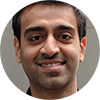##### 15:30 - 16:00 | Secure Software Leasing and Implications to Quantum Copy-Protection and Obfuscation

Authors: Gorjan Alagic, Prabhanjan Ananth, Zvika Brakerski, Yfke Dulek, Rolando La Placa and Christian Schaffner

Abstract

Affiliations: QuICS | UC Santa Barbara | Weizmann Institute of Science | QuSoft, University of Amsterdam | MIT | QuSoft, University of Amsterdam

Extended abstract

Abstract
In quantum copy-protection, an adversary who is given a quantum state computing a function f cannot produce two (possibly entangled) quantum states that each individually compute f. No constructions for copy-protection are known in the plain model. We consider a weaker notion, secure software leasing (SSL), where it is only impossible to produce two copies that can both compute f using the honest evaluation algorithm. We show the following: (1) SSL is possible for a subclass of evasive functions, assuming the existence of post-quantum indistinguishability obfuscators and hardness of LWE; (2) SSL is impossible in general, assuming hardness of LWE. The second statement has important implications for existing quantum-cryptographic notions: in particular, it implies the impossibility of quantum copy-protection for arbitrary unlearnable functions, and impossibility of quantum virtual-black-box obfuscation of classical circuits.##### 16:00 - 17:00 | Secure Computation is in MiniQCrypt

Authors: James Bartusek, Andrea Coladangelo, Dakshita Khurana and Fermi Ma // Alex Bredariol Grilo, Huijia Lin, Fang Song and Vinod Vaikuntanathan

Abstract

Affiliations: LIP6, CNRS/Sorbonne Université | University of Washington | Portland State University | MIT // UC Berkeley | UC Berkeley | University of Illinois, Urbana Champaign | Princeton University and NTT Research

Extended abstract

Abstract
MiniQCrypt is a world where quantum-secure one-way functions exist, and quantum communication is possible. We construct an oblivious transfer (OT) protocol in MiniQCrypt that achieves simulation-security against malicious quantum polynomial-time adversaries, building on the foundational work of Bennett, Brassard, Crépeau and Skubiszewska (CRYPTO 1991). Combining the OT protocol with prior works, we obtain secure two-party and multi-party computation protocols also in MiniQCrypt. This is in contrast to the classical world, where it is widely believed that one-way functions alone do not give us OT.

##### 17:00 - 17:30 | Round tables with Alexander Müller-Hermes, Daniel Ranard, Minh Tran, Prabhanjan Ananth, James Bartusek and Alex Bredariol Grilo

At the end of the session, you can meet with the speakers directly. Round tables take place in parallel, each speaker having their own meeting.

### Session 3A

Main stage (A)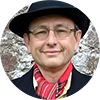19:00 - 19:30
The Hidden Subgroup Problem for Infinite Groups

Authors: Greg Kuperberg

Abstract

Affiliations: Professor of Mathematics and Computer Science, UC Davis, USA

Extended abstract

Abstract
We consider the hidden subgroup problem (HSP) for infinite groups, beyond the celebrated original cases established by Shor and Kitaev. We prove that HSP is NP-hard in the rational numbers ℚ under addition, as well as for normal subgroups of a non-abelian free group F_k. We can show that HSP in the lattice ℤ^k is uSVP-hard with unary encoding of vectors. On the other hand, HSP in ℤ^k with standard binary encoding of vectors can be solved in BQP, uniformly in the dimension, generalizing the Shor-Kitaev algorithm to infinite-index hidden subgroups. HSP in any fixed, finitely generated, virtually abelian subgroup can also be solved in subexponential time using established quantum algorithms for the abelian hidden shift problem.19:30 - 20:00
Quantum algorithms for escaping from saddle points

Authors: Chenyi Zhang, Jiaqi Leng and Tongyang Li

Abstract

Affiliations: Tsinghua University | University of Maryland | Massachusetts Institute of Technology

Extended abstract

Abstract
We initiate the study of quantum algorithms for escaping from saddle points with provable guarantee. Given a function $f:\R^{n}\to\R$, our quantum algorithm outputs an $\epsilon$-approximate local minimum using $\tilde{O}(\log^{2} n/\epsilon^{1.75})$ queries to the quantum evaluation oracle (i.e., the zeroth-order oracle). Compared to the classical state-of-the-art algorithm by Jin et al.~with $\tilde{O}(\log^{6} n/\epsilon^{1.75})$ queries to the gradient oracle (i.e., the first-order oracle), our quantum algorithm is polynomially better in terms of $n$ and matches its complexity in terms of $1/\epsilon$. Our quantum algorithm is built upon two techniques: First, we replace the classical perturbations in gradient descent methods by simulating quantum wave equations, which constitutes the polynomial speedup in $n$ for escaping from saddle points. Second, we show how to use a quantum gradient computation algorithm due to Jordan to replace the classical gradient queries in nonconvex optimization by quantum evaluation queries with the same complexity, extending the same result from convex optimization due to van Apeldoorn et al. and Chakrabarti et al. Finally, we also perform numerical experiments that support our quantum speedup.Authors: Bill Fefferman and Zachary Remscrim // Uma Girish, Ran Raz and Wei Zhan

Abstract

Affiliations: University of Chicago | University of Chicago // Princeton University | Princeton University | Princeton University

Extended abstract

Abstract
A foundational result in the theory of quantum computation, known as the principle of safe storage,'' shows that it is always possible to take a quantum circuit and produce an equivalent circuit that makes all measurements at the end of the computation. While this procedure is time efficient, meaning that it does not introduce a large overhead in the number of gates, it uses extra ancillary qubits, and so is not generally space efficient. It is quite natural to ask whether it is possible to eliminate intermediate measurements without increasing the number of ancillary qubits. We give an affirmative answer to this question by exhibiting a procedure to eliminate all intermediate measurements that is simultaneously space efficient and time efficient. In particular, this shows that the definition of a space-bounded quantum complexity class is robust to allowing or forbidding intermediate measurements. A key component of our approach, which may be of independent interest, involves showing that the well-conditioned versions of many standard linear-algebraic problems may be solved by a quantum computer in less space than seems possible by a classical computer. // We give a quantum logspace algorithm for powering contraction matrices, that is, matrices with spectral norm at most 1. The algorithm gets as an input an arbitrary $n\times n$ contraction matrix $A$, and a parameter $T \leq \mathrm{poly}(n)$ and outputs the entries of $A^T$, up to (arbitrary) polynomially small additive error. The algorithm applies only unitary operators, without intermediate measurements. We show various implications and applications of this result: First, we use this algorithm to show that the class of quantum logspace algorithms with only quantum memory and with intermediate measurements is equivalent to the class of quantum logspace algorithms with only quantum memory without intermediate measurements. This shows that the deferred-measurement principle, a fundamental principle of quantum computing, applies also for quantum logspace algorithms (without classical memory). More generally, we give a quantum algorithm with space $O(S + \log T)$ that takes as an input the description of a quantum algorithm with quantum space $S$ and time $T$, with intermediate measurements (without classical memory), and simulates it unitarily with polynomially small error, without intermediate measurements. Since unitary transformations are reversible (while measurements are irreversible) an interesting aspect of this result is that it shows that any quantum logspace algorithm (without classical memory) can be simulated by a reversible quantum logspace algorithm. This proves a quantum analogue of the result of Lange, McKenzie and Tapp that deterministic logspace is equal to reversible logspace. Finally, we use our results to show non-trivial classical simulations of quantum logspace learning algorithms.

20:30 - 21:00
Round tables with Greg Kuperberg, Jiaqi Leng, Zachary Remscrim and Uma Girish
At the end of the session, you can meet with the speakers directly. Round tables take place in parallel, each speaker having their own meeting.

### Session 3B

Stage BAuthors: Aleksander Kubica and Rafał Demkowicz-Dobrzański

Abstract

Affiliations: AWS Center for Quantum Computing | Faculty of Physics, University of Warsaw

Abstract
We present a simple proof of the approximate Eastin-Knill theorem, which connects the quality of a quantum error-correcting code (QECC) with its ability to achieve a universal set of transversal logical gates. Our derivation employs powerful bounds on the quantum Fisher information in generic quantum metrological protocols to characterize the QECC performance measured in terms of the worst-case entanglement fidelity. The theorem is applicable to a large class of decoherence models, including erasure and depolarizing noise. Our approach is unorthodox, as instead of following the established path of utilizing QECCs to mitigate noise in quantum metrological protocols, we apply methods of quantum metrology to explore the limitations of QECCs.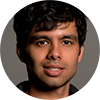19:30 - 20:00
Fusion-based quantum computation

Authors: Sara Bartolucci, Patrick Birchall, Hector Bombin, Hugo Cable, Chris Dawson, Mercedes Gimeno-Segovia, Eric Johnston, Konrad Kieling, Naomi Nickerson, Mihir Pant, Fernando Pastawski, Terry Rudolph and Chris Sparrow

Abstract

Affiliations: PsiQuantum Corp. | PsiQuantum Corp. | PsiQuantum Corp. | PsiQuantum Corp. | PsiQuantum Corp. | PsiQuantum Corp. | PsiQuantum Corp. | PsiQuantum Corp. | PsiQuantum Corp.

Abstract
We introduce fusion based quantum computing (FBQC) - a model of universal quantum computation in which entangling measurements, called fusions, are performed on the qubits of small constant sized entangled resource states. We introduce a stabilizer formalism for analyzing fault tolerance and computation in these schemes. This framework naturally captures the error structure that arises in certain physical systems, such as linear optics. FBQC can offer significant architectural simplifications, enabling hardware made up of many identical modules, and reducing classical processing requirements. We present two pedagogical examples of fault-tolerant schemes constructed in this framework and numerically evaluate their threshold under a general error model with measurement erasure and error, and a linear-optical error model with fusion failure and photon loss. In these schemes, fusion failures, as well as errors, are directly dealt with by the quantum error correction protocol. We find that tailoring the fault-tolerance framework to the physical system allows the scheme to have a higher threshold than schemes reported in literature. We present a ballistic scheme which can tolerate a 10.3% probability of suffering photon loss in each fusion.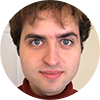Authors: Oscar Higgott and Nikolas Breuckmann

Abstract

Affiliations: University College London | University College London

Abstract
We introduce a technique that uses gauge fixing to significantly improve the quantum error correcting performance of subsystem codes. By changing the order in which check operators are measured, valuable additional information can be gained, and we introduce a new method for decoding which uses this information to improve performance. Applied to the subsystem toric code with three-qubit check operators, we increase the threshold under circuit-level depolarising noise from 0.67% to 0.81%. The threshold increases further under a circuit-level noise model with small finite bias, up to 2.22% for infinite bias. Furthermore, we construct families of finite-rate subsystem LDPC codes with three-qubit check operators and optimal-depth parity-check measurement schedules. To the best of our knowledge, these finite-rate subsystem codes outperform all known codes at circuit-level depolarising error rates as high as 0.2%, where they have a qubit overhead that is 4.3× lower than the most efficient version of the surface code and 5.1× lower than the subsystem toric code. Their threshold and pseudo-threshold exceeds 0.42% for circuit-level depolarising noise, increasing to 2.4% under infinite bias using gauge fixing.

20:30 - 21:00
Round tables with Aleksander Kubica, Mihir Pant and Oscar Higgott
At the end of the session, you can meet with the speakers directly. Round tables take place in parallel, each speaker having their own meeting.

### Session 3C

Stage C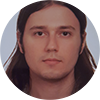19:00 - 19:30
Hamiltonian simulation in the low energy subspace

Authors: Burak Sahinoglu and Rolando Somma

Abstract

Affiliations: Los Alamos National Laboratory | Los Alamos National Laboratory

Extended abstract

Abstract
We study the problem of simulating the dynamics of spin systems when the initial state is supported on a subspace of low energy of a Hamiltonian $H$. This is a central problem in physics with vast applications in many-body systems and beyond, where the interesting physics takes place in the low-energy sector. We analyze error bounds induced by product formulas that approximate the evolution operator and show that these bounds depend on an effective low-energy norm of $H$. We find improvements over the best previous complexities of product formulas that apply to the general case, and these improvements are more significant for long evolution times that scale with the system size and/or small approximation errors. To obtain these improvements, we prove novel exponentially-decaying upper bounds on the leakage to high-energy subspaces due to the product formula. Our results provide a path to a systematic study of Hamiltonian simulation at low energies, which will be required to push quantum simulation closer to reality.Authors: Hakop Pashayan, Oliver Reardon-Smith, Kamil Korzekwa and Stephen Bartlett

Abstract

Affiliations: Institute for Quantum Computing, University of Waterloo ; Perimeter Institute | Jagiellonian University | Jagiellonian University | The University of Sydney

Extended abstract

Abstract
We present two classical algorithms for the simulation of universal quantum circuits on n qubits constructed from c instances of Clifford gates and t arbitrary-angle Z-rotation gates such as T gates. Our algorithms complement each other by performing best in different parameter regimes. The Estimate algorithm produces an additive precision estimate of the Born rule probability of a chosen measurement outcome with the only source of run-time inefficiency being a linear dependence on the stabilizer extent (which scales like ≈1.17^t for T gates). Our algorithm is state-of-the-art for this task: as an example, in approximately 25 hours (on a standard desktop computer), we estimated the Born rule probability to within an additive error of 0.03, for a 50 qubit, 60 non-Clifford gate quantum circuit with more than 2000 Clifford gates. The Compute algorithm calculates the probability of a chosen measurement outcome to machine precision with run-time O(2^(t−r) (t−r)t) where r is an efficiently computable, circuit-specific quantity. With high probability, r is very close to min{t,n−w} for random circuits with many Clifford gates, where w is the number of measured qubits. Compute can be effective in surprisingly challenging parameter regimes, e.g., we can randomly sample Clifford+T circuits with n=55, w=5, c=10^5 and t=80 T-gates, and then compute the Born rule probability with a run-time consistently less than 104 seconds using a single core of a standard desktop computer. We provide a C+Python implementation of our algorithms.Authors: Matthew Coudron and Nolan Coble

Abstract

Affiliations: University of Maryland Computer Science/NIST | University of Maryland Mathematics

Extended abstract

Abstract
We present a classical algorithm that, for any geometrically-local, constant-depth quantum circuit $C$, and any bit string $x \in \{0,1\}^n$, can compute the quantity $|\bra{0^{\otimes n}}C \ket{x}|^2$ to within any inverse-polynomial additive error in quasi-polynomial time. It is known that it is $\#P$-hard to compute this same quantity to within $2^{-n^2}$ additive error \cite{Mov19}. The previous best known algorithm for this problem used $O(2^{n^{1/3}}\poly(1/\epsilon))$ time to compute probabilities to within additive error $\epsilon$ \cite{BGM19}. Notably, the \cite{BGM19} paper included an elegant polynomial time algorithm for the same estimation task with 2D circuits, which makes a novel use of 1D Matrix Product States carefully tailored to the 2D geometry of the circuit in question. Surprisingly, it is not clear that it is possible to extend this use of MPS to address the case of 3D circuits in polynomial time. This raises a natural question as to whether the computational complexity of the 3D problem might be drastically higher than that of the 2D problem. In this work we address this question by exhibiting a quasi-polynomial time algorithm for the 3D case. In order to surpass the technical barriers encountered by previously known techniques we are forced to pursue a novel approach: Constructing a recursive sub-division of the given 3D circuit using carefully designed block-encodings.

20:30 - 21:00
Round table with Burak Sahinoglu, Hakop Pashayan and Matthew Coudron
At the end of the session, you can meet with the speakers directly. Round tables take place in parallel, each speaker having their own meeting.

##### 21:00 - 21:40 | Quantum Quiz

Team up with QIP participants from around the globe to take part in this quantum quiz, with serious and funny questions, all related to quantum science!

JOIN the Quiz in the MeetAnyway space, in the Social Activities tab (scroll down).

Accept privacy?